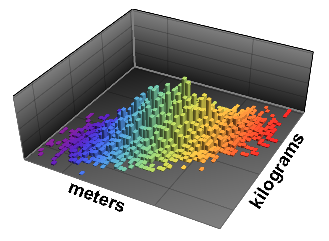# Quantities in Probability & StatisticsMathematica Version 11 supports quantities across the probability and statistics functionality, fully automating conversion, propagation, and transformations - from data to models to correct interpretation.

## Key Features

 Support for quantities across the probability and statistics framework. » Support for quantities in descriptive statistics. » Support for quantities in statistical visualization. » Support for quantities in hypothesis tests. » Support for quantities in time series and other data representations. » Use units to represent a distribution of quantities. » Use quantity parameters in parametric distributions. Use quantity data to build nonparametric distributions. Use quantity formulas and parameters in derived distributions. Use quantities for computing probabilities and expectations.